# Curating the dataset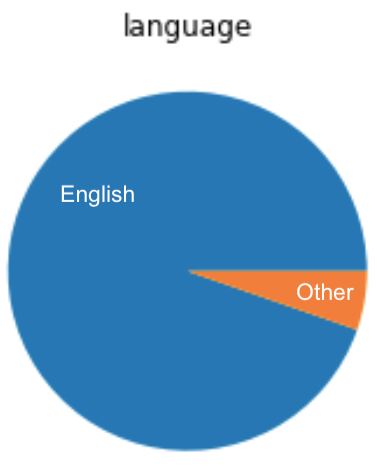Figure 1: Data distribution of(a) numerical data (b) genres and (c) language

# Discussion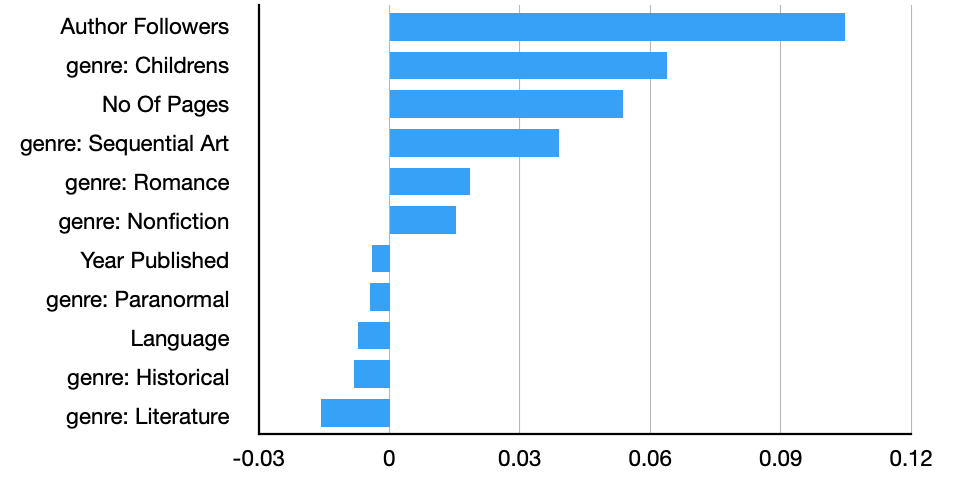Figure 9: Weights of features in linear regression for predicting rating from numerical data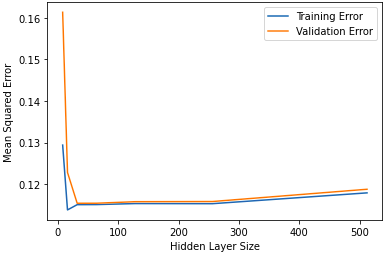Figure 10: Change in MSE on increasing the number of hidden units in the ANN for predicting rating from summary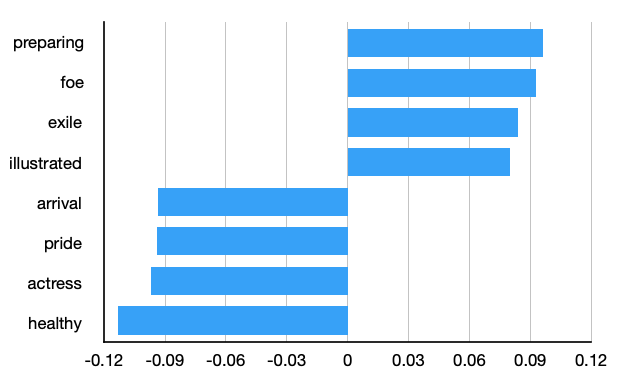Figure 11: Weights of some words in linear regression on Summary for predicting the rating of a book

# Final Model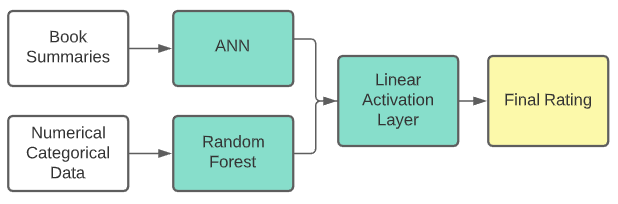Figure 12: Final model architecture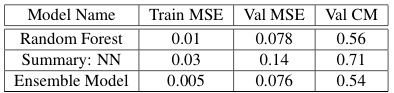Table 5: Performance of the final model as compared to the individual models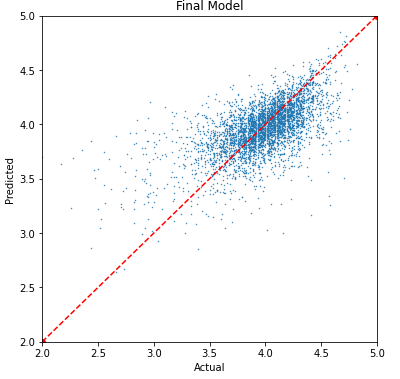Figure 11: Scatterplot of actual vs predicted rating for the final model on testing data

--

--